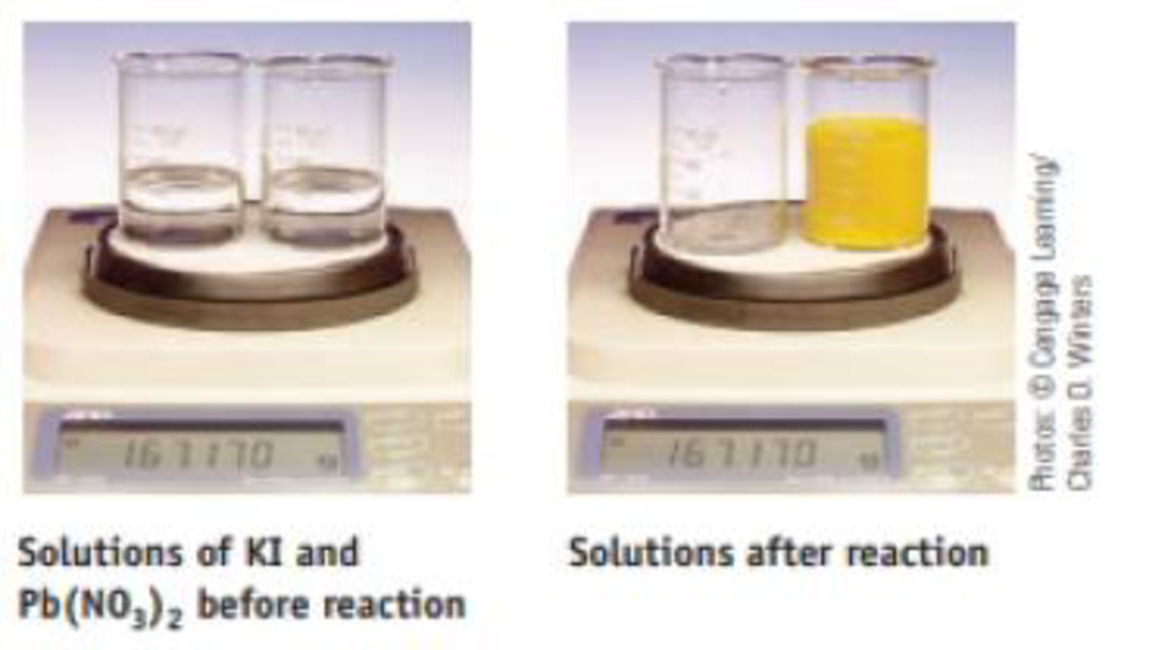Chapter 4, Problem 135SCQ

Chapter
Section
Textbook Problem

Two beakers sit on a balance; the total mass is 167.170 g. One beaker contains a solution of KI; the other contains a solution of Pb(NO3)2. When the solution in one beaker is poured completely into the other, the following reaction occurs:2 KI(aq) + Pb(NO3)2(aq) → 2 KNO3(aq) + PbI2(s)What is the total mass of the beakers and solutions after reaction? Explain completely.

Interpretation Introduction

Interpretation:

The total mass of the beakers and solutions after the given reaction has to be determined and explained.

Concept introduction:

• For chemical reaction balanced chemical reaction equation written in accordance with the Law of conservation of mass.
• Law of conservation of mass states that for a reaction total mass of the reactant and product must be equal.
Explanation

The balanced chemical reaction equation for the given reaction is,

2KI(aq)+Pb(NO3)2(aq)2KNO3<

Still sussing out bartleby?

Check out a sample textbook solution.

See a sample solution

The Solution to Your Study Problems

Bartleby provides explanations to thousands of textbook problems written by our experts, many with advanced degrees!

Get Started